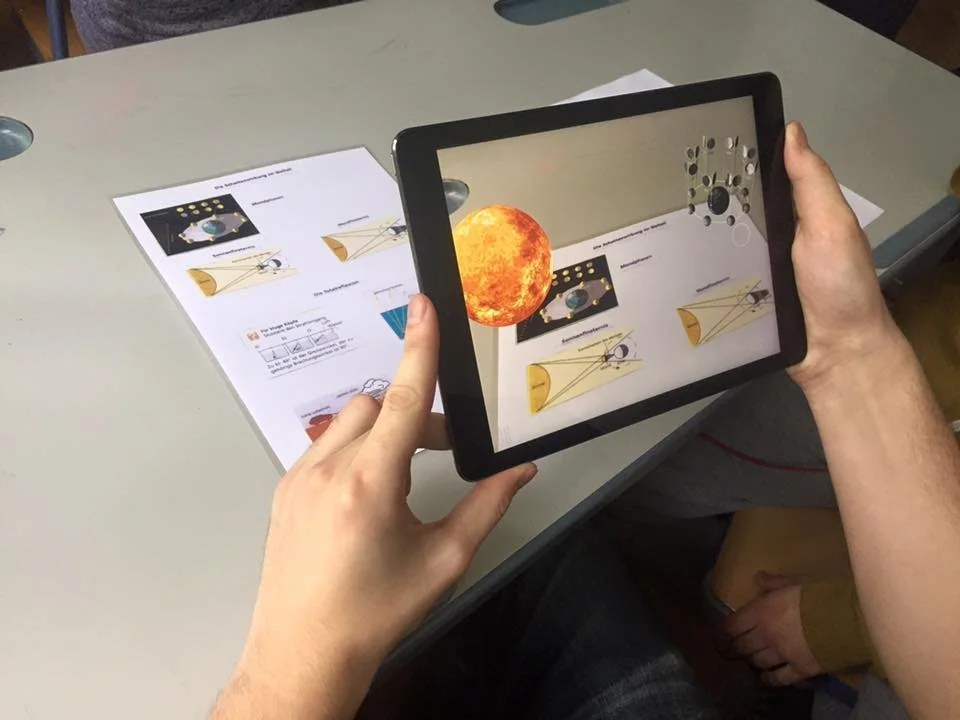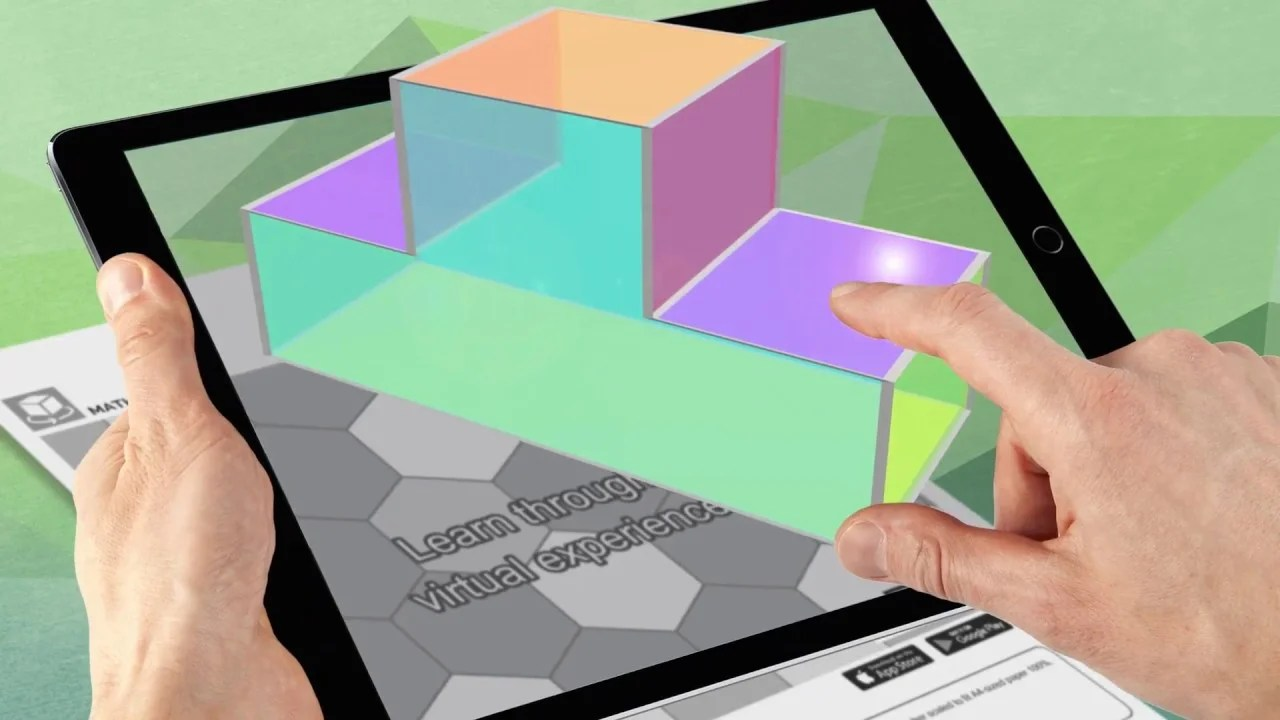# Step Up Your Math Game – Augmented Matrix Solver• September 15, 2023Are you a math enthusiast looking for a way to up your game? Have you ever encountered a complex problem requiring an augmented matrix solver? If so, then this blog post is for you! Here, you will learn how to tackle challenging equations using an augmented matrix solver easily. From linear algebra to algebraic equations, an augmented matrix solver can help you understand how to solve complex mathematical problems quickly and accurately. Keep reading to learn more about using an augmented matrix solver to become a math whiz.

## What is an Augmented Matrix Solver?

Are you tired of spending countless hours trying to solve complex equations by hand? Well, say goodbye to those days of frustration because an augmented matrix solver is here to save the day! But what exactly is an augmented matrix solver?

An augmented matrix solver is a powerful tool in linear algebra that allows you to solve systems of linear equations in a simplified and efficient manner. It takes a system of equations and represents it as an augmented matrix, essentially a rectangular grid of numbers. By manipulating this matrix using various operations, such as row operations, you can quickly solve for the variables in your equations.

This tool is handy when dealing with larger systems of equations or when the equations are particularly complex. It eliminates the need for tedious manual calculations, allowing you to focus on understanding the underlying concepts and finding solutions quickly.

So, whether you’re a math student, a researcher, or just a curious math enthusiast, an augmented matrix solver is an invaluable tool that will elevate your problem-solving skills to new heights. Stay tuned to learn more about how it works and how to use it effectively.

## How Does it Work?Now that you have a basic understanding of an augmented matrix solver let’s dive into how it works. The key concept behind an augmented matrix solver is to manipulate the matrix using row operations to solve for the variables in your system of equations.

To start, you’ll need to input your equations into the solver. This can be done by entering the coefficients and constants into a matrix format. Once your augmented matrix is set up, you can apply row operations to simplify the matrix and solve for the variables.

The most common row operations include swapping rows, multiplying a row by a scalar, and adding or subtracting rows. By performing these operations strategically, you can transform the matrix into a row-echelon or reduced-echelon form.

Once your augmented matrix is in row-echelon or reduced row-echelon form, you can easily read off the solutions to your system of equations. Each pivot position in the matrix corresponds to a leading variable, while any free variables can be set to arbitrary values.

With practice, you’ll become more efficient at using an augmented matrix solver to tackle even the most complex systems of equations. It’s a powerful tool to elevate your problem-solving skills and help you become a math whiz. So, roll up your sleeves and get ready to solve equations like a pro!

### Solving Complex Systems with Augmented Matrices

Solving complex systems of equations can be daunting, but with an augmented matrix solver, it becomes a breeze. An augmented matrix solver is a powerful tool that allows you to tackle even the most challenging systems of equations easily.

When faced with a complex system of equations, you can input the coefficients and constants into the augmented matrix solver. This matrix will then undergo row operations, such as swapping, multiplying a row by a scalar, or adding or subtracting rows. By strategically performing these operations, you can transform the matrix into a simplified form known as a row-echelon or reduced row-echelon structure.

Once your augmented matrix is in this simplified form, the solutions to your system of equations can be easily read. Each pivot position in the matrix corresponds to a leading variable, while any free variables can be assigned arbitrary values.

Using an augmented matrix solver, you can solve complex equations quickly and accurately. It’s a valuable tool that will soon enhance your problem-solving skills and make you a math whiz.

### Advanced Techniques for Augmented Matrix SolvingNow that you have a good grasp of the basics of an augmented matrix solver let’s explore some advanced techniques that can take your matrix-solving skills to the next level. These techniques will enable you to handle more complex systems of equations easily.

One advanced technique is the use of pivoting. Pivoting involves selecting a non-zero entry in a column as a pivot and using it to eliminate other entries in the queue. This technique is beneficial when dealing with systems that have significant coefficients or thresholds with many decimal places.

Another technique is back substitution. This method is used when the augmented matrix is in reduced row-echelon form and involves working backward to find the values of the variables. By starting with the bottom row and solving for each variable one at a time, you can efficiently obtain the solutions.

Finally, remember partial pivoting. This technique involves selecting the most significant entry in a column as the pivot, ensuring numerical stability and reducing the likelihood of round-off errors.

By incorporating these advanced techniques into your augmented matrix-solving process, you can tackle even the most challenging systems of equations confidently and accurately. Happy solving!

### Tips and Tricks for Using an Augmented Matrix Solver

Are you ready to take your augmented matrix-solving skills to the next level? Here are some tips and tricks to help you get the most out of your augmented matrix solver.

1. Practice, practice, practice: Like any tool, the more you use an augmented matrix solver, the better you’ll use it effectively. Take the time to solve various systems of equations using your solver to gain confidence and improve your problem-solving skills.
2. Understand the problem: Before diving into solving a complex system of equations, take a moment to understand the issue. Break it into smaller parts and identify patterns or relationships that can help simplify the process.
3. Use row operations strategically: When applying row operations, be strategic about the processes you choose. Swap rows only when necessary, multiply rows by scalars that simplify calculations, and carefully add or subtract rows to eliminate variables.
4. Keep track of your steps: As you manipulate the augmented matrix, keeping track of the row operations you perform is essential. This will help you avoid mistakes and allow you to backtrack if needed.
5. Verify your solutions: After obtaining solutions using the augmented matrix, verifying them by substituting them back into the original equations is always a good idea. This step will help ensure the accuracy of your results.

With these tips and tricks in your toolkit, you’ll be well-equipped to tackle even the most challenging systems of equations using an augmented matrix solver. So, roll up your sleeves, put your skills to the test, and become a master problem solver in no time. Happy matrix solving!

#### Maximizing Efficiency with Augmented Matrix Solver ShortcutsIf you want to take your augmented matrix-solving skills to the next level and maximize your efficiency, then this section is for you! While an augmented matrix solver is already a powerful tool on its own, you can take a few shortcuts to speed up your problem-solving process even further.

Firstly, familiarize yourself with familiar patterns and relationships in systems of equations. By recognizing these patterns, you can apply specific row operations to simplify the augmented matrix more quickly. This saves you time and effort, allowing you to arrive at the solutions faster.

Secondly, use technology to your advantage. There are numerous online resources and software programs available that offer augmented matrix solvers. Utilizing these tools can automate specific steps in the solving process and reduce the risk of errors.

Finally, practice mental math and calculations. The more comfortable you become with mental arithmetic, the less you’ll need to rely on calculators or external resources. This will significantly increase your speed and efficiency using an augmented matrix.

By incorporating these shortcuts into your problem-solving routine, you’ll become a true master of augmented matrix solving.

### Why The Fusion Of Augmented Reality, NFT and Metaverse Is The Trend For 2023?

Download our free report today that shows you exactly how you could bring in the flavour of augmented reality into your business. Real use cases and exact step by step strategy that can be followed to make sure you don’t miss out this wave!### Why The Fusion Of Augmented Reality, NFT and Metaverse Is The Trend For 2023?

Download our free report today that shows you exactly how you could bring in the flavour of augmented reality into your business. Real use cases and exact step by step strategy that can be followed to make sure you don’t miss out this wave!